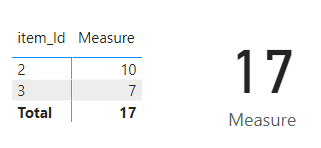cancel
Showing results for
Did you mean:Frequent Visitor

## Sum a colum if the key is present on another table DAX

Hello everyone,

I have an item table:

item_id   price

01           5

02          10

03            7

And I have an event table:

event_id     item_id

grty           02

bfgz          03

fdbx          03

azrq          02

One item can be affected by multiple events.

I would like to have a measure that will sum the total price of all items that are present in the event table.

In this example, that would be item 02 and 03. Therefore the total price would be 17.

Can anyone kindly help me with this DAX problem?

Lucas

1 ACCEPTED SOLUTIONSuper User

@lucasrm , Try one of the two. if first one joined

calculate(sum(Table[price]), filter(event, not(isblank(event[item_id]))))

or

calculate(sum(Table[price]), filter(Table, Table[item_id] in allselected(event[item_id])))

4 REPLIES 4Super User

@lucasrm in this case first you need to create a relationship between two tables like belowthen below is output you are looking forlet me know if it helps you. attached pbix file for reference.

Proud to be a Super User!Frequent Visitor

Hello @negi007 !

Thanks for the help but unfortunately that was not what I was looking for. I had already the two tables linked. I actually needed a measure so I could calculate totals and etc.

I took your pbix file and used the DAX from @amitchandak and it worked!

Here's the result I was looking for:Thanks anyway for the help!Super User

@lucasrm , Try one of the two. if first one joined

calculate(sum(Table[price]), filter(event, not(isblank(event[item_id]))))

or

calculate(sum(Table[price]), filter(Table, Table[item_id] in allselected(event[item_id])))Frequent Visitor

Thanks @amitchandak , it worked great!

I tried something similar before but I was afraid that this type of DAX would sum repeatedly the price for each occurence of item in the event table. I was wrong, it worked perfectly!